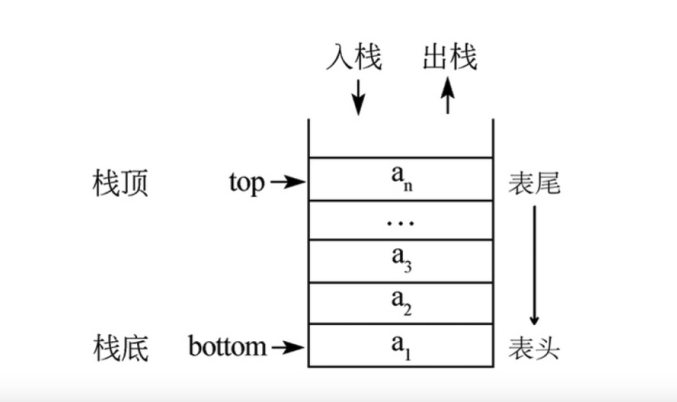Python 数据结构之栈的实现BOB 2020-11-30 14:48:05
• 在其它设备中阅读本文章
•## 栈的概念## 栈的操作

• push(item)：进栈（向栈顶添加元素）
• pop()：出栈（删除栈顶元素）
• top()：查看栈顶元素
• empty()：判断栈是否为空

## Python 实现栈

class Stack:
def __init__(self):
self.stack = []

def push(self, item):
self.stack.append(item)

def pop(self):
if self.empty():
return None
else:
return self.stack.pop()

def top(self):
if self.empty():
return None
else:
return self.stack[-1]

def empty(self):
return len(self.stack) == 0


## 栈的简单应用：括号匹配问题

()[]{}：匹配；{[()]}：匹配；({}]：不匹配；()]：不匹配；({)}：不匹配

• 遇到左小括号 (，执行进栈操作；
• 遇到右小括号 )，判断此时栈顶是否为左小括号 (，是则让左小括号 ( 出栈，此时栈为空;
• 遇到左中括号 [，执行进栈操作；
• 遇到左大括号 {，执行进栈操作；
• 遇到右大括号 }，判断此时栈顶是否为左大括号 {，是则让左大括号 { 出栈，此时栈为空；
• 遇到右中括号 ]，判断此时栈顶是否为左中括号 [，是则让左中括号 [ 出栈，此时栈为空；
• 判断最终的栈是否为空，是则表示匹配，不是则表示不匹配。其中第 ② ⑤ ⑥ 步中，若判断为不是，则直接表示不匹配。

Python 代码实现：

class Stack:
def __init__(self):
self.stack = []

def push(self, item):
self.stack.append(item)

def pop(self):
if self.empty():
return None
else:
return self.stack.pop()

def top(self):
if self.empty():
return None
else:
return self.stack[-1]

def empty(self):
return len(self.stack) == 0

def brackets_match(s):
match_dict = {'}': '{', ']': "[", ')': '('}
stack = Stack()
for ch in s:
if ch in ['(', '[', '{']:    # 如果为左括号，则执行进栈操作
stack.push(ch)
else:                        # 如果为右括号
if stack.empty():        # 如果栈为空，则不匹配，即多了一个右括号，没有左括号匹配
return False
elif stack.top() == match_dict[ch]:  # 如果栈顶的元素为对应的左括号，则让栈顶出栈
stack.pop()
else:                    # 如果栈顶元素不是对应的左括号，则不匹配
return False
if stack.empty():                # 最后的栈如果为空，则匹配，否则不匹配
return True
else:
return False

print(brackets_match('[{()}(){()}[]({}){}]'))
print(brackets_match('()[{}]'))
print(brackets_match('({)}'))
print(brackets_match('[]}'))


True
True
False
False


## 栈的简单应用：倒序输出一组元素

class Stack:
def __init__(self):
self.stack = []

def push(self, item):
self.stack.append(item)

def pop(self):
if self.empty():
return None
else:
return self.stack.pop()

def top(self):
if self.empty():
return None
else:
return self.stack[-1]

def empty(self):
return len(self.stack) == 0

def reverse_list(s):
stack = Stack()
for ch in s:
stack.push(ch)
new_list = []
while not stack.empty():
new_list.append(stack.pop())
return new_list

print(reverse_list(['A', 'B', 'C', 'D', 'E']))


['E', 'D', 'C', 'B', 'A']